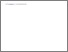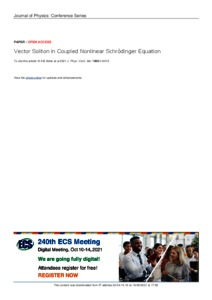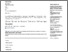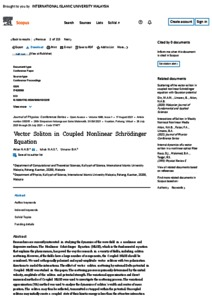Vector soliton in coupled nonlinear Schrödinger equation

Busul Aklan, Nor Amirah and Ishak, N A S and Umarov, Bakhram A. (2021) Vector soliton in coupled nonlinear Schrödinger equation. In: 28th Simposium Kebangsaan Sains Matematik, SKSM 2021, 28-29 July 2021, Virtual.Preview
PDF - Published VersionPreview
PDF (SCOPUS) - Supplemental Material

Abstract

Researchers are currently interested in studying the dynamics of the wave field in a nonlinear and dispersive medium. The Nonlinear Schrödinger Equation (NLSE), which is the fundamental equation that explains the phenomenon, has paved the way for research in a variety of fields, including soliton scattering. However, if the fields have a large number of components,the Coupled NLSE should be considered. We used orthogonally polarised and equal-amplitude vector solitons with two polarization directions to model the interactions. The effect of vector soliton scattering by external Delta potential in Coupled NLSE was studied in this paper. The scattering process is primarily determined by the initial velocity, amplitude of the soliton and potential strength. The variational approximation and direct numerical methods of Coupled NLSE were used to investigate the scattering process. The variational approximation (VA) method was used to analyse the dynamics of soliton’s width and center of mass position. The soliton may thus be reflected, transmitted or trapped within the potential. Uncoupled solitons may initially create a coupled state if their kinetic energy is less than the attractive interaction potential between solitons, but once their velocity surpasses the critical velocity, the soliton will easily pass through each other. To validate the approximation, a direct numerical simulation of CNLSE was performed. The results of the VA method and direct numerical simulation of Coupled NLSE are in good agreement when the parameters for both solutions are set to the same value. The initial velocity, potential strength and soliton amplitude play a role in the scattering of the vector soliton with Delta potential.

Item Type: Conference or Workshop Item (Plenary Papers) Vector soliton; coupled nonlinear Schrödinger equation; delta potential; nonlinear equation; scattering; variational method; numerical method. Q Science > QA MathematicsQ Science > QA Mathematics > QA297 Numerical AnalysisQ Science > QC Physics Kulliyyah of Science > Department of Computational and Theoretical Sciences Dr Nor Amirah Mohd Busul Aklan 06 Oct 2021 15:18 06 Oct 2021 15:18 http://irep.iium.edu.my/id/eprint/92722View Item